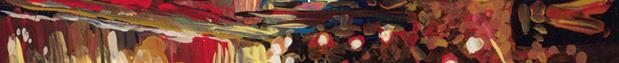The Dictionary of the Climate Debate (DCD)

 initial value problem Definition: The mathematics' term for a problem where you start with a system that has a particular set of conditions at one particular moment in time and then compute how the system will evolve from there. Predicting the weather is an example of this type of problem. Notes: 1. This is in contrast to boundary value problems, where you define a set of unchanging conditions (e.g., the physics of sunlight) and then compute the unchanging average of the weather that these conditions determine. Modelling the climate is an example of this type of problem. 2. To model climate change, you have to combine these two approaches, the resulting software is called a general circulation model.

If you have any comments or criticisms,
please use the box below to let me know.

E-Mail: